Year 7 Interactive Maths - Second Edition

## Bearings

A directional compass is shown below.  It is used to find a direction or bearing .The four main directions of a compass are known as cardinal points.  They are north (N), east (E), south (S) and west (W).  Sometimes, the half-cardinal points of north-east (NE), north-west (NW), south-east (SE) and south-west (SW) are shown on the compass.  The above compass shows degree measurements from 0° to 360° in 10° intervals with:

• north representing 0° or 360°
• east representing 90°
• south representing 180°
• west representing 270°

When using a directional compass, hold the compass so that the point marked north points directly away from you.  Note that the magnetic needle always points to the north.

### Bearing

The true bearing to a point is the angle measured in degrees in a clockwise direction from the north line.  We will refer to the true bearing simply as the bearing.For example, the bearing of point P is 065º which is the number of degrees in the angle measured in a clockwise direction from the north line to the line joining the centre of the compass at O with the point P (i.e. OP).

The bearing of point Q is 300º which is the number of degrees in the angle measured in a clockwise direction from the north line to the line joining the centre of the compass at O with the point Q (i.e. OQ).

###### Note:

The bearing of a point is the number of degrees in the angle measured in a clockwise direction from the north line to the line joining the centre of the compass with the point.

A bearing is used to represent the direction of one point relative to another point.

For example, the bearing of A from B is 065º.  The bearing of B from A is 245º.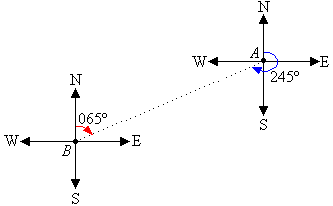###### Note:
• Three figures are used to give bearings.
• All bearings are measured in a horizontal plane.

#### Example 10

State the bearing of the point P in each of the following diagrams:##### Solution:

a.  Mark the angle in a clockwise direction by indicating the turn between the north line and the line joining the centre of the compass to the point P.The bearing of point P is 048°.

b.  Mark the angle in a clockwise direction by indicating the turn between the north line and the line joining the centre of the compass to the point P.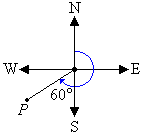The cardinal point S corresponds to 180°.  It is clear from the diagram that the required angle is 60° larger than 180°.  So, the angle measured in a clockwise direction from the north line to the line joining the centre of the compass to point P is 180° + 60° = 240°.

So, the bearing of point P is 240°.

c.  Mark the angle in a clockwise direction by indicating the turn between the north line and the line joining the centre of the compass to the point P.The cardinal point S corresponds to 180°.  It is clear from the diagram that the required angle is 40° less than 180°.  So, the angle measured in a clockwise direction from the north line to the line joining the centre of the compass to point P is 180° 40° = 140°.

So, the bearing of point P is 140°.

d.  Mark the angle in a clockwise direction by indicating the turn between the north line and the line joining the centre of the compass to the point P.The cardinal point W corresponds to 270°.  It is clear from the diagram that the required angle is 20° larger than 270°.  So, the angle measured in a clockwise direction from the north line to the line joining the centre of the compass to point P is 270° + 20° = 290°.

So, the bearing of point P is 290°.

### Direction

The conventional bearing of a point is stated as the number of degrees east or west of the north-south line.  We will refer to the conventional bearing simply as the direction.

To state the direction of a point, write:

• N or S which is determined by the angle being measured
• the angle between the north or south line and the point, measured in degrees
• E or W which is determined by the location of the point relative to the north-south lineE.g. In the above diagram, the direction of:

• A from O is N30ºE.
• B from O is N60ºW.
• C from O is S70ºE.
• D from O is S80ºW.
###### Note:

N30ºE means the direction is 30º east of north.

#### Example 11

Describe each of the following bearings as directions.
a.  076°
b.  150°
c.  225°
d.  290°

##### Solution:

a.  The position of a point P on a bearing of 076° is shown in the following diagram.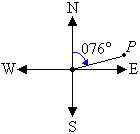The position of the point P is 76° east of north.  So, the direction is N76°E.

b.  The position of a point P on a bearing of 150° is shown in the following diagram.The position of the point P is 180° 150° = 30° east of south.  So, the direction is S30°E.

c.  The position of a point P on a bearing of 225° is shown in the following diagram.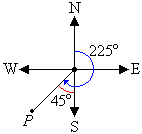The position of the point P is 225° 180° = 45° west of south.  So, the direction is S45°W.

d.  The position of a point P on a bearing of 290° is shown in the following diagram.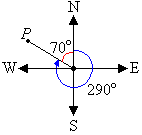The position of the point P is 360° 290° = 70° west of north.  So, the direction is N70°W.